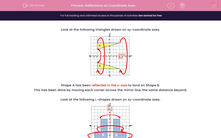# Reflections on Coordinate Axes

In this worksheet, students reflect shapes on a coordinate grid.Key stage:  KS 2

Curriculum topic:   Maths and Numerical Reasoning

Curriculum subtopic:   Transformations

Difficulty level:#### Worksheet Overview

Look at the following triangles drawn on xy-coordinate axes.Shape A has been reflected in the x-axis to land on Shape B.

This has been done by moving each corner across the mirror line, the same distance beyond.

Look at the following L-shapes drawn on xy-coordinate axes.Shape A has been reflected in the y-axis to land on Shape B.

This has been done by moving each corner across the mirror line, the same distance beyond.

Example

Look at the triangles on this coordinate grid.

On which triangle does Triangle D land when it is reflected in the y-axis?